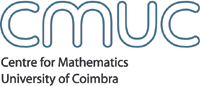Algebra and CombinatoricsMembersSeminarsEventsPublicationsdetails .: download Author(s) Olga Azenhas; Ricardo Mamede; TitleMatrix realizations of pairs of Young tableaux, keys and shuffles AbstractLet $\sigma\in{\mathcal S}_t$ and ${\mathcal H}_{\sigma}$ an associated key [6, 7, 12]. Given the pair of Young tableaux $({\mathcal T},{\mathcal H}_{\sigma})$, where ${\mathcal T}$ is a skew-tableau with the same evaluation as ${\mathcal H}_{\sigma}$, we consider the problem of a matrix realization of the pair $({\mathcal T},{\mathcal H}_{\sigma})$, over a local principal ideal domain [2, 3, 4]. It has been shown that, when $\sigma$ is the identity , the reverse permutation in ${\mathcal S}_t$ , or any permutation in ${\mathcal S}_3$ , $({\mathcal T},{\mathcal H}_{\sigma})$ has a matrix realization if and only if the word of $\mathcal T$ is in the plactic class of ${\mathcal H}_{\sigma}$. Here, we extend the only if condition of this result to any $\sigma\in{\mathcal S}_t$, $t\geq 1$. For a sequence of nonnegative integers $(m_1,\ldots,m_t)$ and $1\leq i\leq t$, this amounts to give a criterion which extends a word congruent with the key of evaluation $(m_1,\ldots,m_{i-1},m_{i+1},\ldots,m_t)$, to one congruent with the key of evaluation $(m_1,\ldots,m_t)$. For the identity, the reverse permutation in ${\mathcal S}_t$, or an arbitrary permutation in ${\mathcal S}_3$, the plactic class of an associated key may be described as shuffles of their rows . For $t\geq 4$, this is no longer true for an arbitrary permutation $\sigma\in{\mathcal S}_t$. Instead, we find that shuffling together the rows of a key always leads to a congruent word. The permutations $\sigma\in{\mathcal S}_t$ whose plactic classes of associated keys are described by shuffling together their rows, are identified. For $\sigma\in{\mathcal S}_4$, we show that we may describe the plactic class of any associated key, in terms of shuffling, by adding, in those cases where the rows of the key are not enough, just one single word. JournalSéminaire Lotharingien de Combinatoire Volume53 Year2006 IssueB53h Page(s)22pp. CMUC Apartado 3008, 3001 - 454 Coimbra, Portugal T:+351 239 791 150 F:+351 239 793 069 cmuc@mat.uc.pt - developed by Flor de Utopia